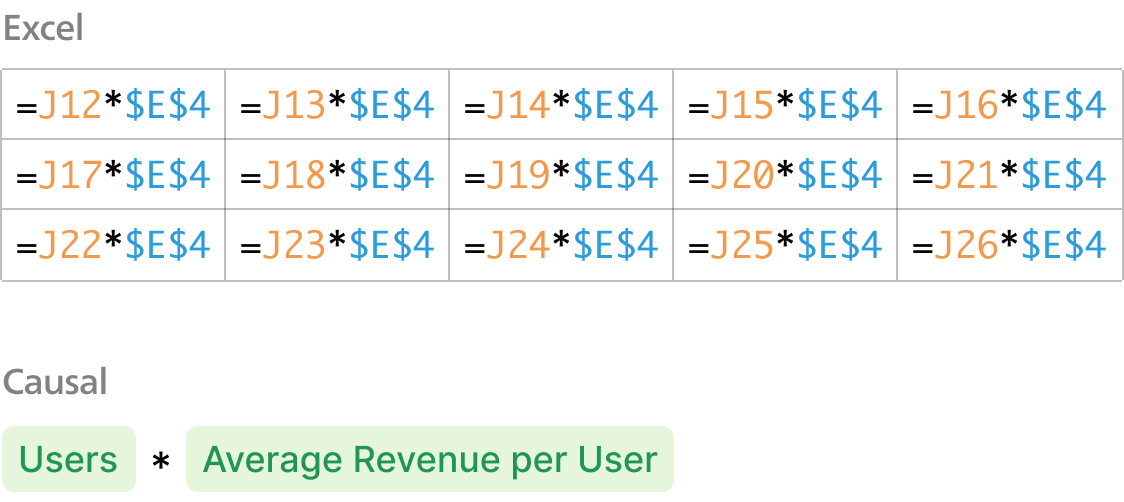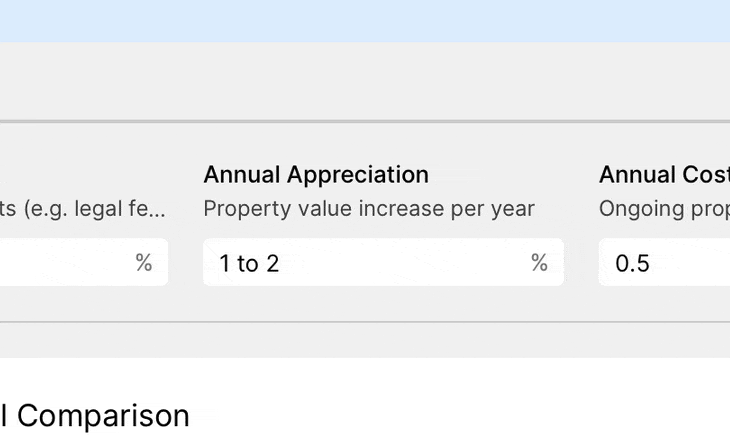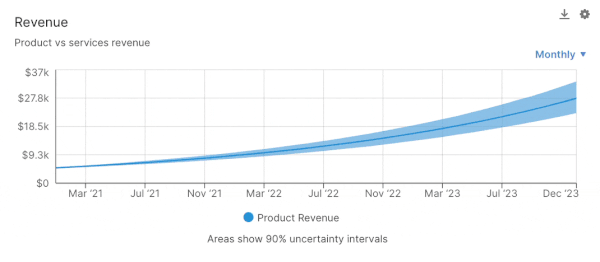How to Calculate Quick Ratio in Salesforce

What is Quick Ratio?

Quick Ratio is a liquidity ratio that measures a company's ability to pay short-term obligations.

The Quick Ratio is calculated by dividing a company's cash, cash equivalents, and short-term investments by its current liabilities.

The Quick Ratio is a more conservative measure of liquidity than the Current Ratio because it excludes inventory from current assets.

The Quick Ratio is also known as the Acid-Test Ratio.

How do you calculate Quick Ratio in Salesforce?

It can be difficult to calculate Quick Ratio directly inside of Salesforce; that's where Causal comes in.

Causal is a modelling tool which lets you build models on top of your Salesforce data. You simply connect Causal to your Salesforce account, and then you can build formulae in Causal to calculate your Quick Ratio.

What is Causal?

Causal lets you build models effortlessly and share them with interactive, visual dashboards that everyone will understand.

In Causal, you build your models out of variables, which you can then link together in simple plain-English formulae to calculate metrics like Quick Ratio. This makes your models easy to understand and quick to build, so you can spend minutes, not days, on your models.When you're done, you can share the link to your model with stakeholders. They'll be able to view your model's outputs in a visual dashboard, rather than a jumble of tabs and complex formulae. The dashboards are interactive, letting viewers tweak your assumptions to see how they affect the model's outputs.Causal lets you add visuals in a single click, letting you plot out graphs and distributions for metrics like Quick Ratio.data

Thanks! We'll be in touch soon.
Oops! Something went wrong while submitting the form.